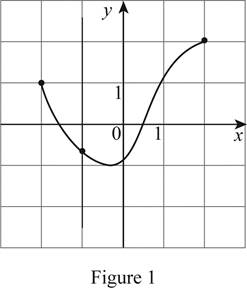# The curve is a graph of a function of x . If so, find the domain and range of the function.### Single Variable Calculus: Concepts...

4th Edition
James Stewart
Publisher: Cengage Learning
ISBN: 9781337687805### Single Variable Calculus: Concepts...

4th Edition
James Stewart
Publisher: Cengage Learning
ISBN: 9781337687805

#### Solutions

Chapter 1.1, Problem 6E
To determine

## The curve is a graph of a function of x. If so, find the domain and range of the function.

Expert Solution

The curve is the graph of a function of x.

### Explanation of Solution

Vertical line test states that the curve in the xy coordinate plane is the graph of the function of x if and only if a vertical line intersects the curve at exactly one point.

Perform the vertical line test for the given graph.

Draw a vertical line such that it passes through the curve as shown below in Figure 1.It is observed from Figure 1 that the vertical line intersects the curve exactly at one point. Therefore, the curve is the graph of a function of x.

Since, the domain of a function is the set of all possible x values of the graph, the domain of the function is 2x2 .

Since, the range of a function is the set of all possible y values of the graph, the range of the function is 1y2 .

### Have a homework question?

Subscribe to bartleby learn! Ask subject matter experts 30 homework questions each month. Plus, you’ll have access to millions of step-by-step textbook answers!